# Probability Darts

5th graders can practise calculating the probability that a dart will hit a given sector of a dart board using these fun probability worksheets.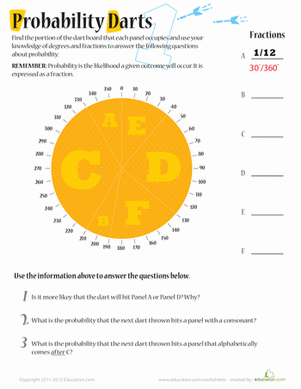### Probability Darts 1

Kids will practise with fractions and degrees in this probability worksheet. Help your kid figure out the probability of a dart landing in different places.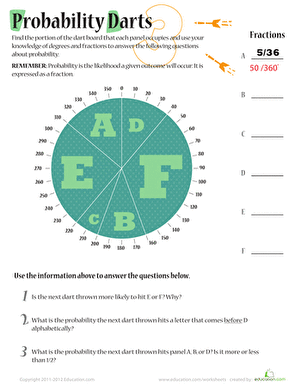### Probability Darts 3

Build on fraction maths skills and work with degrees to get probability practise for your middle schooler with this maths worksheet series.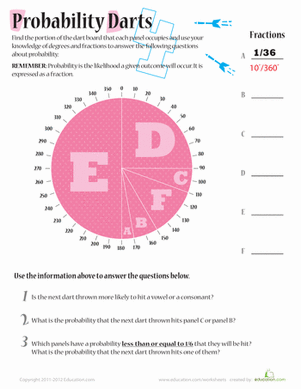### Probability Darts 4

Building on maths skills with fractions, addition, and degrees, kids will figure out how much of the dart board each panel takes up then answer questions.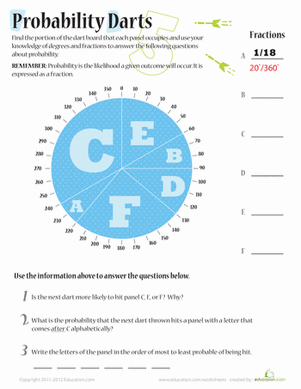### Probability Darts 5

Build on fraction maths skills and working with degrees up to 360° get probability practise for your middle schooler with this maths worksheet series.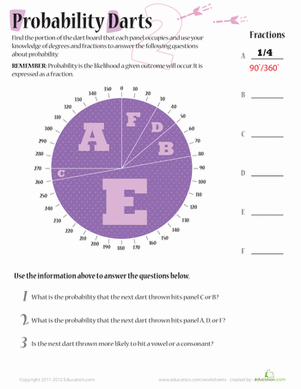### Probability Darts 2

Build on fraction maths skills and working with degrees to get probability practise for your middle schooler with this maths worksheet series.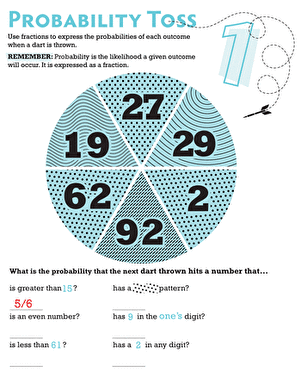### Probability Toss 1

What are the chances that a dart will land on an even number on a dart board? Find out in this sixth grade probability worksheet!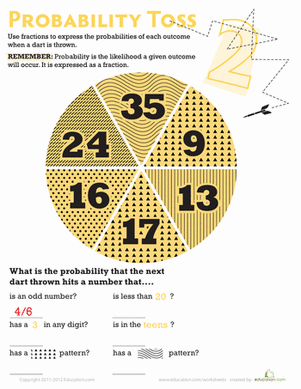### Probability Toss 2

Boost your child's maths know-how! What are the odds a dart would land on one of the given sections of this circle?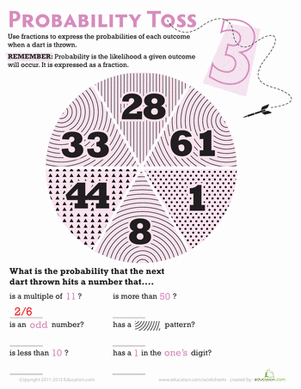### Probability Toss 3

If your sixth grader likes darts, then he'll love this probability worksheet! This dart board themed worksheet introduces your child to simple probability.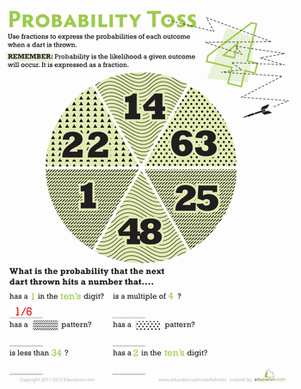### Probability Toss 4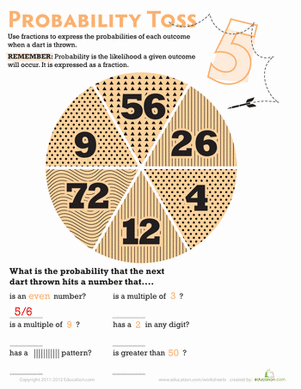### Probability Toss 5

Sixth graders will practise probability in this dart board themed worksheet. Your kid will calculate the probability of a dart hitting a certain kind of number.

Create new collection

0

### New Collection>

0Items

What could we do to improve Education.com?